Submitted Successfully!
Thank you for your contribution! You can also upload a video entry or images related to this topic.
Ver. Summary Created by Modification Content Size Created at Operation
1 handwiki -- 1398 2022-12-02 01:41:03 |
2 format correction Meta information modification 1398 2022-12-02 07:53:53 |

Do you have a full video?

# Confirm

Are you sure to Delete?
Cite
Liu, H. Strain Rate Tensor. Encyclopedia. Available online: https://encyclopedia.pub/entry/37778 (accessed on 28 November 2023).
Liu H. Strain Rate Tensor. Encyclopedia. Available at: https://encyclopedia.pub/entry/37778. Accessed November 28, 2023.
Liu, Handwiki. "Strain Rate Tensor" Encyclopedia, https://encyclopedia.pub/entry/37778 (accessed November 28, 2023).
Liu, H.(2022, December 02). Strain Rate Tensor. In Encyclopedia. https://encyclopedia.pub/entry/37778
Liu, Handwiki. "Strain Rate Tensor." Encyclopedia. Web. 02 December, 2022.
Strain Rate Tensor

In continuum mechanics, the strain rate tensor is a physical quantity that describes the rate of change of the deformation of a material in the neighborhood of a certain point, at a certain moment of time. It can be defined as the derivative of the strain tensor with respect to time, or as the symmetric component of the gradient (derivative with respect to position) of the flow velocity. The strain rate tensor is a purely kinematic concept that describes the macroscopic motion of the material. Therefore, it does not depend on the nature of the material, or on the forces and stresses that may be acting on it; and it applies to any continuous medium, whether solid, liquid or gas. On the other hand, for any fluid except superfluids, any gradual change in its deformation (i.e. a non-zero strain rate tensor) gives rise to viscous forces in its interior, due to friction between adjacent fluid elements, that tend to oppose that change. At any point in the fluid, these stresses can be described by a viscous stress tensor that is, almost always, completely determined by the strain rate tensor and by certain intrinsic properties of the fluid at that point. Viscous stress also occur in solids, in addition to the elastic stress observed in static deformation; when it is too large to be ignored, the material is said to be viscoelastic.

physical quantity strain rate tensor viscoelastic

## 1. Definition

Consider a material body, solid or fluid, that is flowing and/or moving in space. Let v be the velocity field within the body; that is, a smooth function from 3 × ℝ such that v(p,t) is the macroscopic velocity of the material that is passing through the point p at time t.

The velocity v(p + r,t) at a point displaced from p by a small vector r can be written as a Taylor series:

$\displaystyle{ \mathbf{v}(\mathbf{p}+\mathbf{r},t) = \mathbf{v}(\mathbf{p},t)+(\nabla \mathbf{v})(\mathbf{p},t)(\mathbf{r})+\text{higher order terms}, }$

where v the gradient of the velocity field, understood as a linear map that takes a displacement vector r to the corresponding change in the velocity.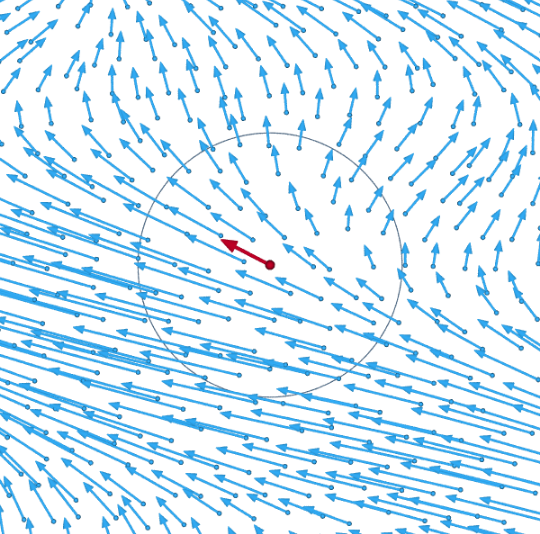Total field v(p + r). https://handwiki.org/wiki/index.php?curid=1418285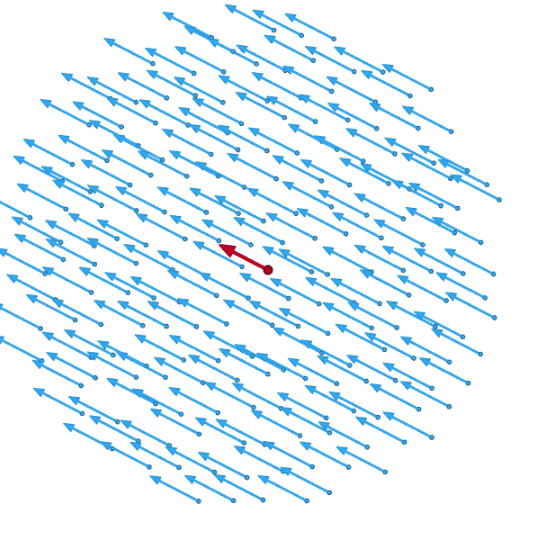Constant part v(p). https://handwiki.org/wiki/index.php?curid=1418285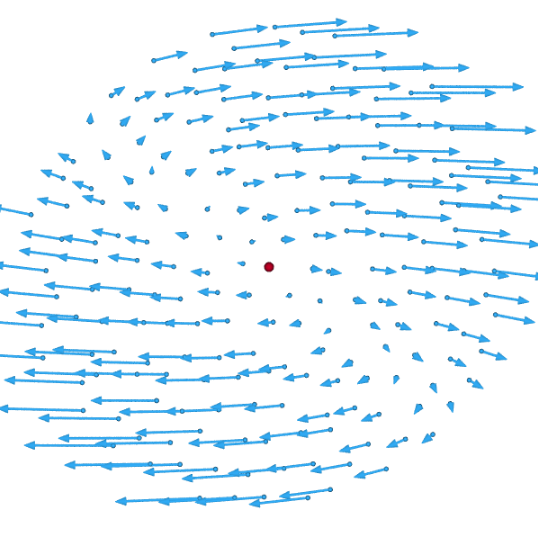Linear part (∇v)(p,t)(r). https://handwiki.org/wiki/index.php?curid=1425457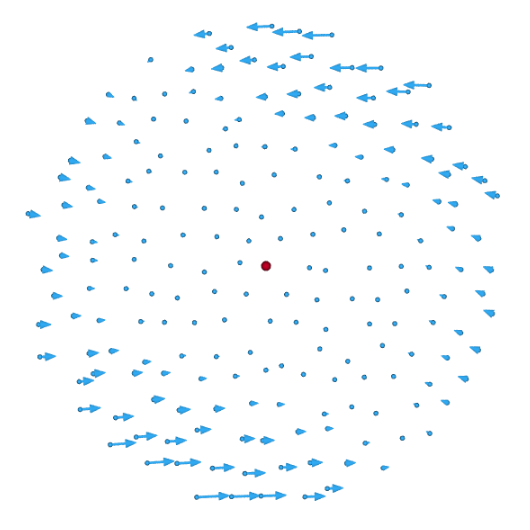Non-linear residual. https://handwiki.org/wiki/index.php?curid=1806859
The velocity field v(p + r,t) of an arbitrary flow around a point p (red dot), at some instant t, and the terms of its first-order Taylor approximation about p. The third component of the velocity (out of the screen) is assumed to be zero everywhere.

In an arbitrary reference frame, v is related to the Jacobian matrix of the field, namely in 3 dimensions it is the 3 × 3 matrix

$\displaystyle{ (\nabla \mathbf{v})^\mathrm{T} = \begin{bmatrix} \displaystyle{\partial_1 v_1} & \displaystyle{\partial_2 v_1} & \displaystyle{\partial_3 v_1}\\ \displaystyle{\partial_1 v_2} & \displaystyle{\partial_2 v_2} & \displaystyle{\partial_3 v_2}\\ \displaystyle{\partial_1 v_3} & \displaystyle{\partial_2 v_3} & \displaystyle{\partial_3 v_3} \end{bmatrix} = \mathbf{J} . }$

where vi is the component of v parallel to axis i and jf denotes the partial derivative of a function f with respect to the space coordinate xj. Note that J is a function of p and t.

In this coordinate system, the Taylor approximation for the velocity near p is

$\displaystyle{ v_i(\mathbf{p} + \mathbf{r},t) = v_i(\mathbf{p},t) + \sum_j J_{i j}(\mathbf{p},t) r_j = v_i(\mathbf{p},t) + \sum_j \partial_j v_i(\mathbf{p},t) r_j; }$

or simply

$\displaystyle{ \mathbf{v}(\mathbf{p} + \mathbf{r},t) = \mathbf{v}(\mathbf{p},t) + \mathbf{J}(\mathbf{p},t) \mathbf{r} }$

if v and r are viewed as 3 × 1 matrices.

### 1.1. Symmetric and Antisymmetric Parts

The symmetric part E(p,t)(r) (strain rate) of the linear term of the example flow. https://handwiki.org/wiki/index.php?curid=1857507
The antisymmetric part R(p,t)(r) (rotation) of the linear term. https://handwiki.org/wiki/index.php?curid=1385458

Any matrix can be decomposed into the sum of a symmetric matrix and an antisymmetric matrix. Applying this to the Jacobian matrix J = (∇v)T with symmetric and antisymmetric components E and R respectively:

$\displaystyle{ \mathbf{E} = \tfrac12\left( \mathbf{J} + \mathbf{J}^\mathrm{T}\right) \qquad \mathbf{R} = \tfrac12\left(\mathbf{ J} - \mathbf{J}^\mathrm{T}\right) }$

That is,

$\displaystyle{ E_{i j} = \tfrac12( \partial_j v_i + \partial_i v_j ) \qquad R_{i j} = \tfrac12( \partial_j v_i - \partial_i v_j) }$

This decomposition is independent of coordinate system, and so has physical significance. Then the velocity field may be approximated as

$\displaystyle{ \mathbf{v}(\mathbf{p} + \mathbf{r},t) \approx \mathbf{v}(\mathbf{p},t) + \mathbf{E}(\mathbf{p},t)(\mathbf{r}) + \mathbf{R}(\mathbf{p},t)(\mathbf{r}), }$

that is,

\displaystyle{ \begin{align} v_i(\mathbf{p} + \mathbf{r},t) &= v_i(\mathbf{p},t) + \sum_j E_{i j}(\mathbf{p},t) r_j + \sum_j R_{i j}(\mathbf{p},t) r_j\\ &= v_i(\mathbf{p},t) + \tfrac12\sum_j \big(\partial_j v_i(\mathbf{p},t)+\partial_i v_j(\mathbf{p},t)\big)r_j + \tfrac12\sum_j \big(\partial_j v_i(\mathbf{p},t)-\partial_i v_j(\mathbf{p},t)\big)r_j \end{align} }

The antisymmetric term R represents a rigid-like rotation of the fluid about the point p. Its angular velocity ω is

$\displaystyle{ \omega=\tfrac12 \nabla\times \mathbf{v}= \tfrac12 \begin{bmatrix} \partial_2 v_3-\partial_3 v_2\\ \partial_3 v_1-\partial_1 v_3\\ \partial_1 v_2-\partial_2 v_1 \end{bmatrix}. }$

The product ∇ × v is called the rotational curl of the vector field. A rigid rotation does not change the relative positions of the fluid elements, so the antisymmetric term R of the velocity gradient does not contribute to the rate of change of the deformation. The actual strain rate is therefore described by the symmetric E term, which is the strain rate tensor.

### 1.2. Shear Rate and Compression RateThe scalar part D(p,t)(r) (uniform expansion/compression rate) of the strain rate tensor E(p,t)(r). https://handwiki.org/wiki/index.php?curid=1553684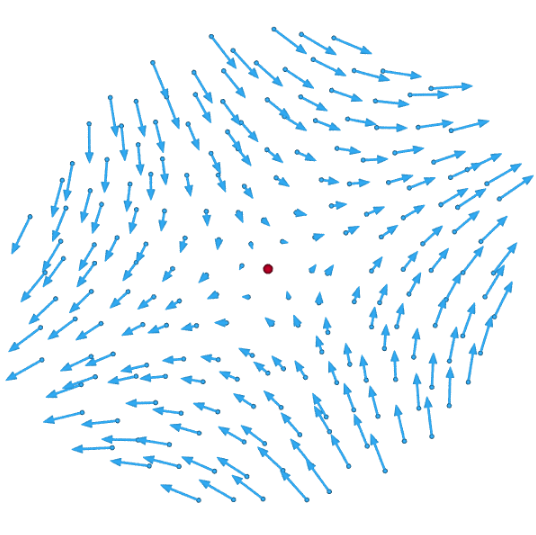The traceless part S(p,t)(r) (shear rate) of the strain rate tensor E(p,t)(r). https://handwiki.org/wiki/index.php?curid=1803999

The symmetric term E of velocity gradient (the rate-of-strain tensor) can be broken down further as the sum of a scalar times the unit tensor, that represents a gradual isotropic expansion or contraction; and a traceless symmetric tensor which represents a gradual shearing deformation, with no change in volume:

$\displaystyle{ \mathbf{E}(\mathbf{p},t)(\mathbf{r}) = \mathbf{D}(\mathbf{p},t)(\mathbf{r}) + \mathbf{S}(\mathbf{p},t)(\mathbf{r}). }$

That is,

$\displaystyle{ E_{ij} = \underbrace{\tfrac13\left(\sum_k\partial_k v_k\right) \delta_{ij}}_{\text{rate-of-expansion tensor} D_{ij}} + \underbrace{\tfrac12\left(\partial_i v_j+\partial_j v_i\right)-\tfrac13\left(\sum_k\partial_k v_k\right) \delta_{ij}}_{\text{rate-of-shear tensor} S_{ij}}, }$

Here δ is the unit tensor, such that δij is 1 if i = j and 0 if ij. This decomposition is independent of the choice of coordinate system, and is therefore physically significant.

The expansion rate tensor is 1/3 of the divergence of the velocity field:

$\displaystyle{ \nabla \cdot \mathbf{v} = \partial_1 v_1 + \partial_2 v_2 + \partial_3 v_3; }$

which is the rate at which the volume of a fixed amount of fluid increases at that point.

The shear rate tensor is represented by a symmetric 3 × 3 matrix, and describes a flow that combines compression and expansion flows along three orthogonal axes, such that there is no change in volume. This type of flow occurs, for example, when a rubber strip is stretched by pulling at the ends, or when honey falls from a spoon as a smooth unbroken stream.

For a two-dimensional flow, the divergence of v has only two terms and quantifies the change in area rather than volume. The factor 1/3 in the expansion rate term should be replaced by 1/2 in that case.

### References

1. Landau, L. D.; Lifshitz, E. M. (1997). Fluid Mechanics (2nd ed.). Butterworth Heinemann. ISBN 0-7506-2767-0.
More
Information
Subjects:
Contributor MDPI registered users' name will be linked to their SciProfiles pages. To register with us, please refer to https://encyclopedia.pub/register :
View Times: 2385
Entry Collection:
Revisions: 2 times (View History)
Update Date: 02 Dec 2022
1000/1000